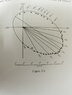# Find the mass of the Sirius system

Ithilrandir
Homework Statement:
The trigonometric parallax of sirius (i.e, the angle subtended at sirius by the radius of the earth's orbit) is 0.378 degrees arc. Using this and data in Fig. 3-2, deduce as best you can the mass M of the sirius system in terms of that of the sun, and
a) assuming that the orbital plane is perpendicular to the line of sight, and
b) allowing for the actual tilt of the orbit.
Is your value in part (b) above an upper or lower limit (or either)?
Relevant Equations:
...
I'll be focusing on mainly trying to find a way to solve part a, as I don't actually know how to account for the tilt.

When they said degrees arc I assume they're just saying degrees, so I found the distance of Earth to sirius to be around 1.50 x 10^8 km(radius of the earth), because of the small angle subtended.

I also found the period to be around 50+ years, by looking at the graph over the years. After that I'm stuck. In order to find the mass, I still need to know the radius of orbit of sirius, but I don't know how to read the graph to find that.

#### Attachments

•IMG_20210128_134711.jpg
31.7 KB · Views: 54
Last edited:

Homework Helper
Gold Member
2022 Award
I found the distance of Earth to sirius to be around 1.50 x 10^8 km(radius of the earth), because of the small angle subtended.
No, the small angle means it is much further away. Do you understand parallax?
Do you understand what a parsec is?

What is "5hus"?

Ithilrandir
No, the small angle means it is much further away. Do you understand parallax?
Do you understand what a parsec is?

What is "5hus"?
The 5hus was a typo which I've amended.

My notion of parallax is in this picture.

Are parsec related to the parallax?

#### Attachments

Homework Helper
Gold Member
2022 Award
My notion of parallax is in this picture.
Ok, so if b is 1.5x108km and the angle labelled π (terrible choice for a variable name) is 0.378 degrees then what is d?
Are parsec related to the parallax?
Yes. Look it up.

Ithilrandir
Ok, so if b is 1.5x108km and the angle labelled π (terrible choice for a variable name) is 0.378 degrees then what is d?

Yes. Look it up.

d = b/tan 0.378.

I've looked up what parsec is. It's the distance from which 1AU subtends at one arc second.

Homework Helper
Gold Member
2022 Award
d = b/tan 0.3
Ok, so what is that in km?
By the way, for such small angles you don't need to bother with the tan function. Just write d = b/(angle in radians).

Ithilrandir
Ok, so what is that in km?
By the way, for such small angles you don't need to bother with the tan function. Just write d = b/(angle in radians).

3.97 x 10^8 km. How does this length relate to the graph in the question?

Homework Helper
Gold Member
2022 Award
The trigonometric parallax of sirius (i.e, the angle subtended at sirius by the radius of the Earth's orbit) is 0.378 degrees arc.
No. The trigonometric parallax of Sirius is not 0.378 degrees of arc. It is 0.378 seconds of arc (often abbreviated to ‘arcsec’). That's a big difference.

Also, note that an expression such as ‘tan 0.378’ is meaningless unless you know what unit is being used for the angle. E.g.
tan(0.378 arcsec)
tan (0.378º)
are completely different values.

By convention, if we leave out the unit we imply that the angle is in radians. So ‘tan 0.378’ would be interpreted as ‘tan(0.378 radians)’.

As a basic skill, you need to learn/understand how to change arc seconds to degrees and to radians. And you need to ensure that the value of an angle entered into a calculator is being entered in the appropriate unit.

As already noted by @haruspex, for small angles (as in this question), the tangent of the angle is, to a very good approximation, equal to the angle in radians.